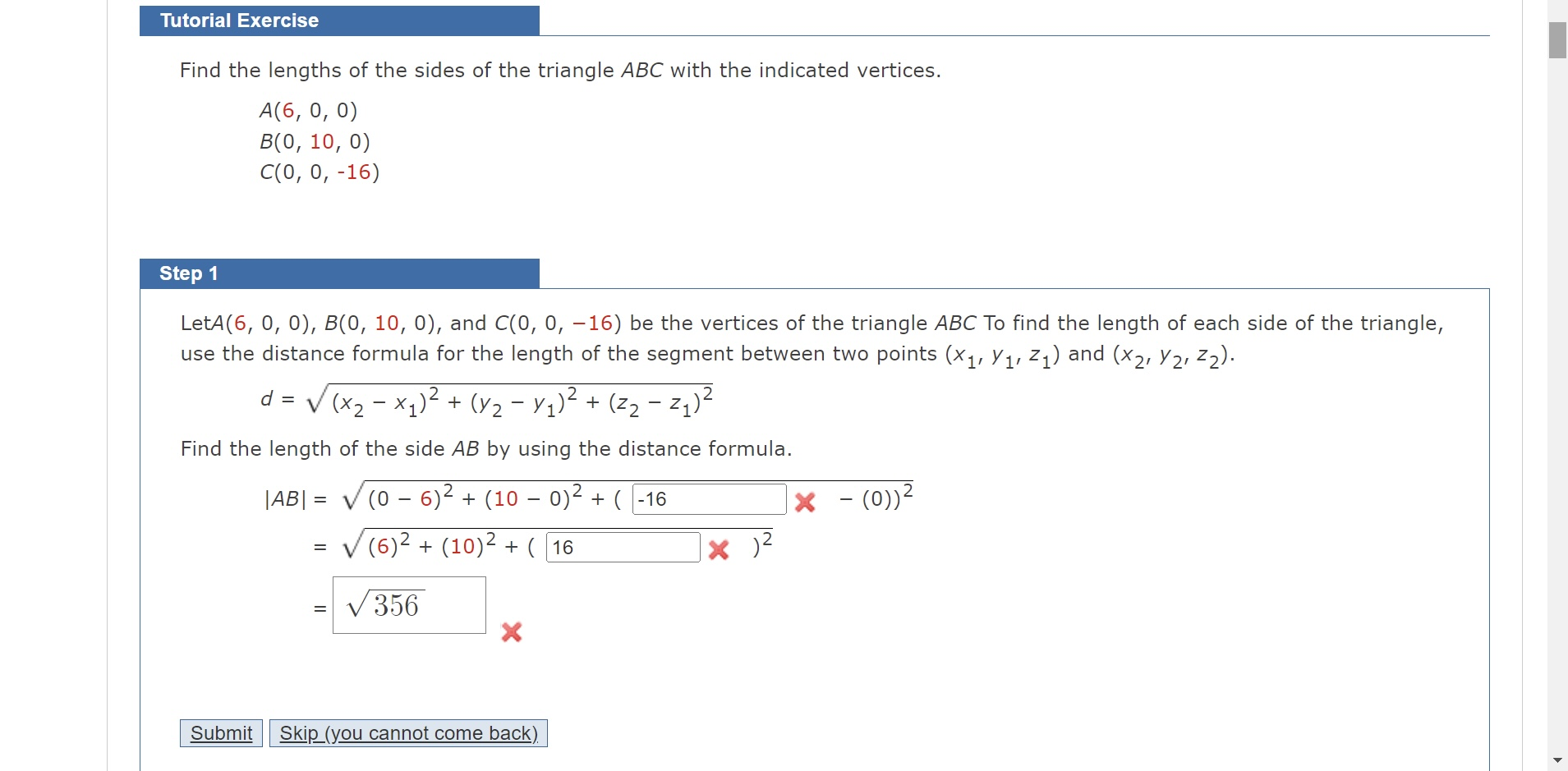Home / Expert Answers / Calculus / find-the-lengths-of-the-sides-of-the-triangle-abc-with-the-indicated-vertices-a-6-0-0-b-0-10-0-c-0-pa628

# (Solved): Find the lengths of the sides of the triangle ABC with the indicated vertices. A(6,0,0)B(0,10,0)C(0 ...Find the lengths of the sides of the triangle with the indicated vertices. Step 1 Let , and be the vertices of the triangle To find the length of each side of the triangle, use the distance formula for the length of the segment between two points and . Find the length of the side by using the distance formula.

We have an Answer from Expert